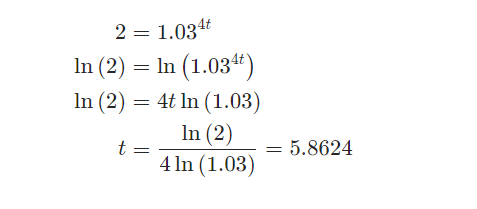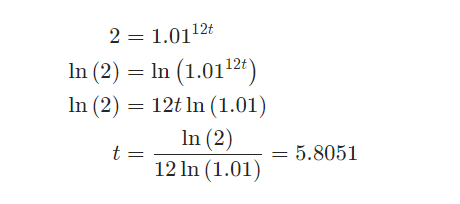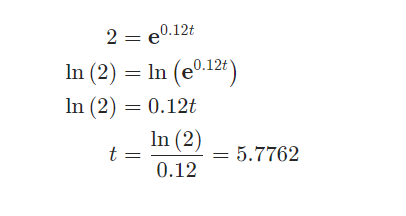### ¿Todavía tienes preguntas de matemáticas?

Pregunte a nuestros tutores expertos
Algebra
Pregunta

2. We are starting with \$5000 and we’re going to put it into an account that earns an annual interest rate of 12%. How long should we leave the money in the account in order to double our money if interest is compounded

quarterly

monthly

continuously

From the problem statement we can see that,

$A = 10000\hspace{0.25in}P = 5000\hspace{0.25in}r = \frac{{12}}{{100}} = 0.12$

Remember that the value of $$r$$ must be given as a decimal, i.e. the percentage divided by 100. Also, for this part we are compounding interest rate quarterly and that means it will compound 4 times per year and so we also then know that,

$m = 4$

Plugging into the equation gives us,

$10000 = 5000{\left( {1 + \frac{{0.12}}{4}} \right)^{4t}} = 5000{\left( {1.03} \right)^{4t}}$

Using the techniques from the Solve Exponential Equations section we can solve for $$t$$.So, we’ll double our money in approximately 5.8624 years.

From the problem statement we can see that,

$A = 10000\hspace{0.25in}P = 5000\hspace{0.25in}r = \frac{{12}}{{100}} = 0.12$

Remember that the value of $$r$$ must be given as a decimal, i.e. the percentage divided by 100. Also, for this part we are compounding interest rate monthly and that means it will compound 12 times per year and so we also then know that,

$m = 12$

Plugging into the equation gives us,

$10000 = 5000{\left( {1 + \frac{{0.12}}{{12}}} \right)^{12t}} = 5000{\left( {1.01} \right)^{12t}}$

Using the techniques from the Solve Exponential Equations section we can solve for $$t$$.So, we’ll double our money in approximately 5.8051 years.

From the problem statement we can see that,

$A = 10000\hspace{0.25in}P = 5000\hspace{0.25in}r = \frac{{12}}{{100}} = 0.12$

Remember that the value of $$r$$ must be given as a decimal, i.e. the percentage divided by 100. For this part we are compounding continuously and so we won’t have an $$m$$ and will be using the other equation.

Plugging into the continuously compounding interest equation gives,

$10000 = 5000{{\bf{e}}^{0.12t}}$

Now, solving this using the techniques from the Solve Exponential Equations section gives,So, we’ll double our money in approximately 5.7762 years.

Solución
View full explanation on CameraMath App.